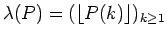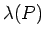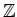Journal of Integer Sequences, Vol. 22 (2019), Article 19.1.1

## A Note on Polynomial Sequences Modulo Integers

### Mohammad Javaheri Department of Mathematics School of Science Siena College Loudonville, NY 12211 USA

Abstract:

We study the uniform distribution of the polynomial sequencemodulo integers, where P(x) is a polynomial with real coefficients. In the nonlinear case, we show thatis uniformly distributed inif and only if P(x) has at least one irrational coefficient other than the constant term. In the case of even degree, we prove a stronger result:intersects every congruence class modulo every integer if and only if P(x) has at least one irrational coefficient other than the constant term.

Full version:  pdf,    dvi,    ps,    latex

Received September 11 2018; revised version received December 16 2018. Published in Journal of Integer Sequences, December 17 2018.